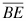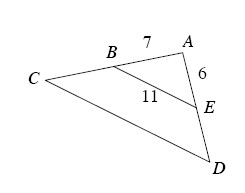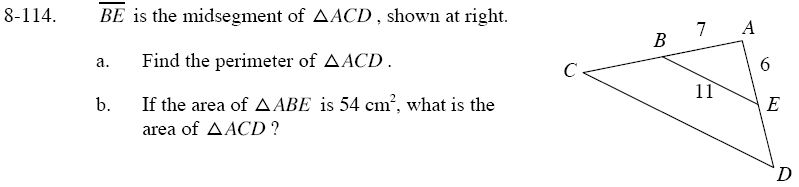Home > GC > Chapter 8 > Lesson 8.3.3 > Problem8-114

8-114.
1.is the midsegment of ΔACD , shown below. Homework Help ✎1. Find the perimeter of ΔACD.

2. If the area of ΔABE is 54 cm2, what is the area of ΔACD ?The perimeter of ΔACD = (2)(the perimeter of ΔABE).
Since BE is a midsegment, the two triangle have a zoom factor of 2, that is why it is multiplied by 2.

The area of ΔACD = (the area of ΔABE)(the zoom factor)².